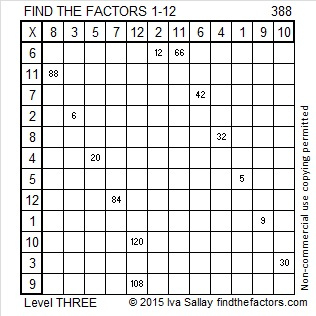# 388 and Level 3

88 is divisible by 4 so 388 is also divisible by 4 and is a composite number. 88 is also divisible by 8, but the third to the last digit is odd, making 388 NOT divisible by 8.Print the puzzles or type the factors on this excel file:12 Factors 2015-02-09

• 388 is a composite number.
• Prime factorization: 388 = 2 x 2 x 97, which can be written 388 = (2^2) x 97
• The exponents in the prime factorization are 2 and 1. Adding one to each and multiplying we get (2 + 1)(1 + 1) = 3 x 2  = 6. Therefore 388 has exactly 6 factors.
• Factors of 388: 1, 2, 4, 97, 194, 388
• Factor pairs: 388 = 1 x 388, 2 x 194, or 4 x 97
• Taking the factor pair with the largest square number factor, we get √388 = (√4)(√97) = 2√97 ≈ 19.698A Logical Approach to FIND THE FACTORS: Find the column or row with two clues and find their common factor. Write the corresponding factors in the factor column (1st column) and factor row (top row).  Because this is a level three puzzle, you have now written a factor at the top of the factor column. Continue to work from the top of the factor column to the bottom, finding factors and filling in the factor column and the factor row one cell at a time as you go.This site uses Akismet to reduce spam. Learn how your comment data is processed.